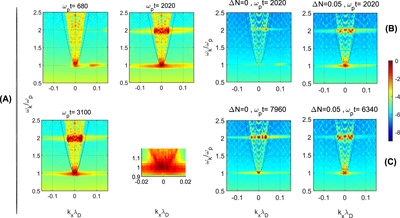Image Details

Choose export citation format:Fundamental Electromagnetic Emissions by a Weak Electron Beam in Solar Wind Plasmas with Density Fluctuations

• Authors: C. Krafft, and P. Savoini

2022 The Astrophysical Journal Letters 924 L24.

• Provider: AAS Journals

Caption: Figure 4.

Dispersion plots of the wave spectral magnetic energy densities log10(∣Bzk(ωk, kx)∣2) as a function of the normalized frequency ωk/ωp and parallel wave vector kxλD, for a homogeneous plasma with ΔN = 0 and a plasma with density fluctuations (ΔN = 0.05). The simulations used are the same as in Figures 23. Panel A: dispersion plots for ΔN = 0.05, at ωpt = 680, 2020, and 3100, computed with the “large” simulation box; a zoom of the frequency wave-number domain 0.9 < ωk/ωp < 1.2 and ∣kxλD∣ < 0.02 is shown for ωpt = 3100 in the bottom right corner. Panel B : dispersion plots obtained using the “smaller” box, at ωpt = 2020, for ΔN = 0 (left) and ΔN = 0.05 (right). Panel C: dispersion plots at asymptotic times for ΔN = 0 (left, ωpt = 7960) and ΔN = 0.05 (right, ωpt = 6340); the “smaller” simulation box is used. The same color bar holds for all plots of panels (A), (B), and (C). All variables are normalized.